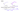## Regularization: Cost Function

Suppose there is an issue of overfitting due to the presence of a large number of features. Consider the hypothesis function $h_\theta(x) = \theta_0 + \theta_1x + \theta_2x^2 + \theta_3x^3 + \theta_4x^4$ and we want to remove the influence of $x^3$ and $x^4$ terms which might be causing overfitting. Without actually getting rid of these features or changing the form of our hypothesis, we can instead modify our cost function.

In order for $x^3$ and $x^4$ terms of the cost function to get close to zero, we will have to reduce the values of $\theta_3$ and $\theta_4$ to near zero. We can rewrite the cost function as:

$J(\theta) = \dfrac{1}{2m} \begin{bmatrix} \displaystyle \sum_{i=1}^m (h_\theta(x^{(i)}) - y^{(i)})^2 + \Big( 1000\cdot\theta_3^2 + 1000 \cdot\theta_4^2 \Big) \end{bmatrix}$We could also regularize all of our theta parameters in a single summation as:

$J(\theta) = \dfrac{1}{2m}\ \begin{bmatrix} \displaystyle \sum_{i=1}^m (h_\theta(x^{(i)}) - y^{(i)})^2 + \lambda \sum_{j=1}^n \theta_j^2 \end{bmatrix}$

The $\lambda$, or lambda, is the regularization parameter. It determines how much the costs of our theta parameters are inflated/deflated. The extra summation term added on the right is called the regularization term. Note that the regularization term does not include $\theta_0$ since we do not want to penalize $\theta_0$.

If the regularization parameter $(\lambda)$ is chosen to be too large, it will deflate all the theta values (except $\theta_0$) to nearly zero and thus smooth out the hypothesis function too much. In this case, the hypothesis function essentially becomes $h_\theta(x) \approx \theta_0$ causing underfitting (high bias).